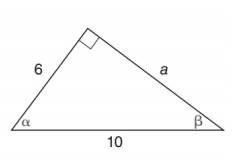Chapter 11.2, Problem 3E### Elementary Geometry for College St...

6th Edition
Daniel C. Alexander + 1 other
ISBN: 9781285195698

#### Solutions

Chapter
Section### Elementary Geometry for College St...

6th Edition
Daniel C. Alexander + 1 other
ISBN: 9781285195698
Textbook Problem
1 views

# In Exercises 1 to 6, find cos α and cos β .To determine

To find:

cosα and cosβ.

Explanation

Consider the following figure,

General formula for cosine ratio is given below,

From the given figure the value 10 is the hypotenuse value since it is opposite to right angle.

Then 6 is the length of the leg adjacent to α and a is the length of leg adjacent to β.

The Pythagorean theorem is given below,

(hypotenuse)2=(lengthofoneleg)2+(lengthofotherleg)2

Substitute the values of hypotenuse=10,lengthofoneleg=6 and lengthofotherleg=a to get the following,

### Still sussing out bartleby?

Check out a sample textbook solution.

See a sample solution

#### The Solution to Your Study Problems

Bartleby provides explanations to thousands of textbook problems written by our experts, many with advanced degrees!

Get Started

#### Find more solutions based on key concepts